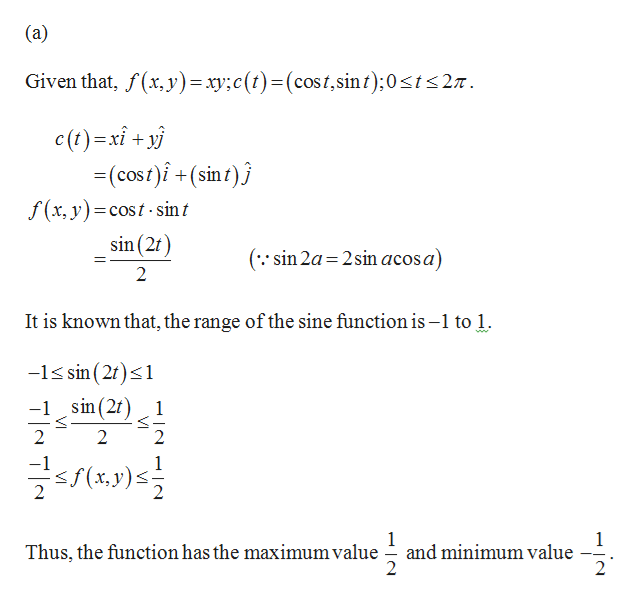# Find the maximum and minimum values attained by the function f along the path c(t).(a) f(x, y) = xy; c(t) = (cos(t), sin(t)); 0 ≤ t ≤ 2π        maximum value __________        minimum value  __________(b) f(x, y) = x2 + y2; c(t) = (cos(t), 8 sin(t)); 0 ≤ t ≤ 2π        maximum value __________        minimum value  __________

Question
218 views
Find the maximum and minimum values attained by the function f along the path c(t).
(a) f(x, y) = xy; c(t) = (cos(t), sin(t)); 0 ≤ t ≤ 2π
maximum value __________
minimum value  __________
(b) f(x, y) = x2 + y2; c(t) = (cos(t), 8 sin(t)); 0 ≤ t ≤ 2π
maximum value __________
minimum value  __________
check_circle

Step 1help_outlineImage Transcriptionclose(a) Given that, f(x, y)=xyc(t)(cost,sin t);0sts27 c(t)xi+y (cost)i+(sinf)j f(x, y)=cost-sin t sin (2f 11 ( sin 2a 2sin acosa) 2 It is known that, the range of the sine function is -1 to 1 -1s sin (2t)s1 -1 sin(2f) 1 2 2 х, у) < 1 and minimum value 2 Thus, the function has the maximum value 2 fullscreen

### Want to see the full answer?

See Solution

#### Want to see this answer and more?

Solutions are written by subject experts who are available 24/7. Questions are typically answered within 1 hour.*

See Solution
*Response times may vary by subject and question.
Tagged in

### Calculus